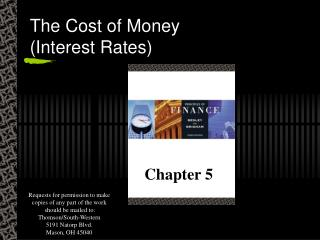# The Cost of Money (Interest Rates) - PowerPoint PPT PresentationDownload PresentationThe Cost of Money (Interest Rates)

The Cost of Money (Interest Rates)Download Presentation## The Cost of Money (Interest Rates)

- - - - - - - - - - - - - - - - - - - - - - - - - - - E N D - - - - - - - - - - - - - - - - - - - - - - - - - - -
##### Presentation Transcript

1. The Cost of Money(Interest Rates) Chapter 5 Requests for permission to make copies of any part of the work should be mailed to: Thomson/South-Western5191 Natorp Blvd.Mason, OH 45040

2. The Cost of Money • Interest rates represent the prices paid to borrow funds • Equity investors expect to receive dividends and capital gains

3. Realized Returns (Yields)

4. Factors that Affect the Cost of Money • Production opportunities • returns available within an economy from investment in productive assets • Time preferences for consumption • the preferences of consumers for current consumption as opposed to saving for future consumption

5. Factors that Affect the Cost of Money • Risk • the chance that a financial asset will not earn the return promised • Inflation • the tendency of prices to increase over time

6. Interest Rates - Supply & Demand for Funds

7. Determinants of Market Interest Rates • Quoted interest rate = k = (k* + IP) + DRP + LP + MRP = kRF + DRP + LP + MRP • k = the quoted or nominal rate • k*= the real risk-free rate of interest • kRF = the quoted, or nominal risk-free rate • IP= inflation premium • DRP= default risk premium • LP= liquidity, or marketability, premium • MRP = maturity risk premium

8. The Real Risk-Free Rate of Interest, k* • The rate of interest that would exist on default-free U. S. Treasury securities if no inflation were expected • Ranges from 2 to 4 percent in the U. S. in recent years

9. Nominal Risk-Free Rate of Interest, kRF • kRF = k* + IP • The rate of interest on a security that is free of all risk, except inflation • Proxied by the T-bill rate or T-bond rate • kRF includes an inflation premium

10. Inflation Premium (IP) • A premium for expected inflation that investors add to the real risk-free rate of return

11. Default Risk Premium (DRP) • Difference between the interest rate on a U. S. Treasury bond and a corporate bond of equal maturity and marketability • Compensates for risk that a borrower will default on a loan

12. Liquidity Premium (LP) • Premium added to the rate on a security if the security cannot be converted to cash on short notice and at close to the original cost

13. Interest Rate Risk • Risk of capital losses to which investors are exposed because of changing interest rates

14. Maturity Risk Premium (MRP) • Premium that reflects the interest rate risk • Bonds with longer maturities have greater interest rate risk • Reinvestment rate risk is greater for short-term bonds

15. Term Structure of Interest Rates • Relationship between yields and maturities of securities • The graph is a yield curve

16. U.S. Treasury Bond Interest Rates

17. Yield Curve • “Normal” Yield Curve • upward sloping yield curve • Inverted (“Abnormal”) Yield Curve • downward sloping yield curve

18. Why Do Yield Curves Differ? • Expectations theory • shape of the yield curve depends on investors’ expectations about future inflation rates • Liquidity preference theory • lenders prefer to make short-term loans borrowers prefer long-term debt

19. Why Do Yield Curves Differ? • Market segmentation theory • each borrower has a preferred maturity and the slope of the yield curve depends on the supply of and demand for funds in the long-term market relative to the short-term market

20. Other Factors That Influence Interest Rate Levels • Federal Reserve policy • Level of the federal budget deficit • Foreign trade balance • Level of business activity

21. Interest Rates and Stock Prices • Higher interest rates increase costs and thus lower a firm’s profits • Interest rates affect the level of economic activity and corporate profits • Interest rates affect investment competition between stocks and bonds

22. End of Chapter 5 The Cost of Money(Interest Rates)